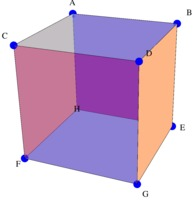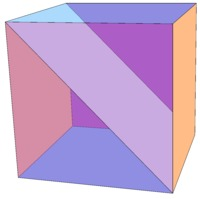# Cube Ninjas!

Alignments to Content Standards: 7.G.A.3

Imagine you are a ninja that can slice solid objects straight through. You have a solid cube in front of you. You are curious about what 2-dimensional shapes are formed when you slice the cube. For example, if you make a slice through the center of the cube that is parallel to one of the faces, the cross section is a square:For each of the following slices, (i) describe using precise mathematical language the shape of the cross section. (ii) draw a diagram showing the cross section of the cube.a. A slice containing edge AC and edge EG b. A slice containing the vertices C, B, and G. C. A slice containing the vertex A, the midpoint of edge EG, and the midpoint of edge FG.

## IM Commentary

The Standards for Mathematical Practice focus on the nature of the learning experiences by attending to the thinking processes and habits of mind that students need to develop in order to attain a deep and flexible understanding of mathematics. Certain tasks lend themselves to the demonstration of specific practices by students. The practices that are observable during exploration of a task depend on how instruction unfolds in the classroom. While it is possible that tasks may be connected to several practices, the commentary will spotlight one practice connection in depth. Possible secondary practice connections may be discussed but not in the same degree of detail.

Students will be engaged in Mathematical Practice Standard 5, â€œUse appropriate tools strategically,â€ by considering the available tools when solving this task and making sound decisions about which and when these tools might be helpful. At the same time, students recognize both the insights to be gained and the limitations of the tool/tools selected.Â  Being able to visualize 2-dimensional shapes within a 3-dimensional object may be very difficult for students. Making tools available will help students approach this task and give them valuable practice in visualizing these types of problems. Appropriate tools include: computer access, paper models, clay, and other tools that allow for the deconstruction of a cube.Â  Mathematical Practice Standard 6, attend to precision, is very integral to this task also. Precision in language is required as students describe the resulting shape of the slice.

## Solution

a. A rectangle.Comment: In Grade 7, students have not had the Pythagorean theorem, so they are not expected to determine that the rectangle has sides of 1 and $\sqrt{2}$. They should, however, note that the diagonal of a face is longer than the edge, hence the cross section is not a square.

b. An equilateral triangle.Comment: Because the three sides of the cross-section are all the diagonals of the faces, they have the same length. As noted in part A, the students are not expected to determine the length to be $\sqrt{2}$, but they are expected to note that all three lengths are the same, hence the triangle is equilateral.

c. An irregular pentagon.Comment: This one is by far the most challenging of the three. A key insight is to figure out where the plane intersects the edges CF and BE. Many people have a great deal of difficulty visualizing the shape. However, students using clay or paper models can figure it out. Although it is not expected for 7th grade students to be able to determine the exact coordinates (it ends up that the intersections are â…” the way up the edge), students can estimate that it is more than halfway, and be able to make an argument that the resulting shape has five sides and not all the sides have the same length, hence it is an irregular pentagon.